# rcs

Calculate and plot radar cross section (RCS) of platform, antenna, or array

Since R2019b

## Syntax

``rcs(object,frequency)``
``rcs(object,frequency,azimuth,elevation)``
``rcs(___,Name=Value)``
``````[rcsval,azimuth,elevation] = rcs(object,frequency)``````
``````[rcsval,azimuth,elevation] = rcs(___,Name=Value)``````

## Description

example

````rcs(object,frequency)` plots the monostatic RCS of the platform, antenna, or array over a specified frequency. To learn more about RCS, see What Is RCS?.```
````rcs(object,frequency,azimuth,elevation)` plots the monostatic RCS of the platform, antenna, or array over a specified frequency for the specified azimuth and elevation angles.```
````rcs(___,Name=Value)` plots the RCS with additional properties specified using one or more Name-Value Arguments.```
``````[rcsval,azimuth,elevation] = rcs(object,frequency)``` returns the RCS value and corresponding azimuth and elevation angles of a platform, antenna, or array at the specified frequency.```
``````[rcsval,azimuth,elevation] = rcs(___,Name=Value)``` returns the RCS value and corresponding azimuth and elevation angles using additional properties specified by one or more name-value arguments.```

## Examples

collapse all

Create a default helix antenna and plot the RCS at 2 GHz.

```ant = helix; rcs(ant,2e9)```Create a default linear array and plot the RCS at 75 MHz in the elevation pane.

```array = linearArray; rcs(array,75e6,0,0:1:360)```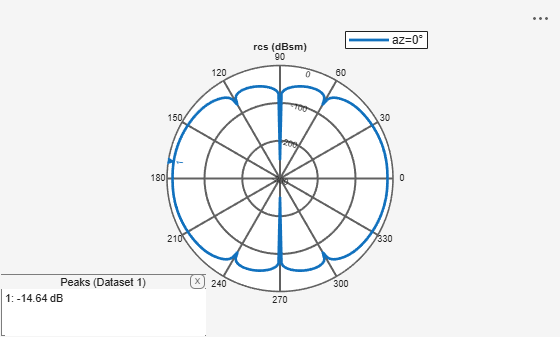Create a reflector-backed dipole and plot the RCS at 1 GHz in the elevation plane at 90 degree azimuth.

```ant = reflector; rcs(ant,1e9,90,0:1:360)```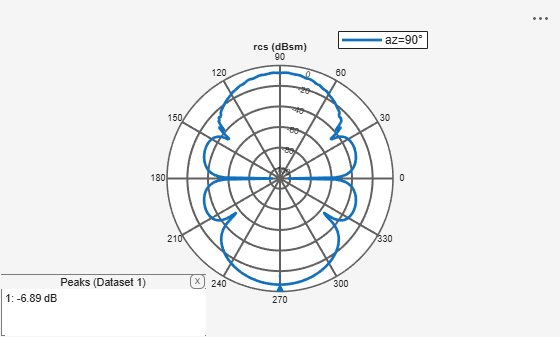Create a tetrahedron platform from an STL file.

```p = platform; p.FileName = "tetrahedra.stl"; p.Units = "m"; figure show(p)```Mesh the platform with edge length of 0.1

```figure mesh(p,MaxEdgeLength=0.1)```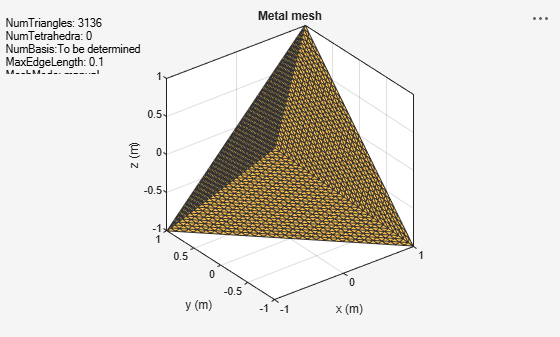Sweep over the elevation with a vertically polarized E-field. Plot the RCS at 700 MHz in the azimuth plane.

```az = 0:1:360; el = 0; figure rcs(p,700e6,az,el)```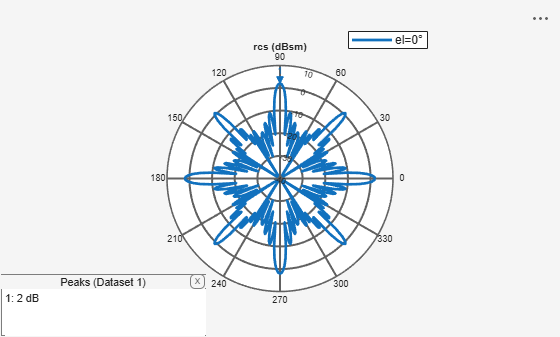Create a corner reflector-backed antenna.

```f = 2e9; c = design(reflectorCorner,750e6);```

Plot the RCS in the elevation plane.

```figure rcs(c,f,0,0:2:360)```Plot the RCS in the azimuth plane.

```figure rcs(c,f,0:2:360,0)```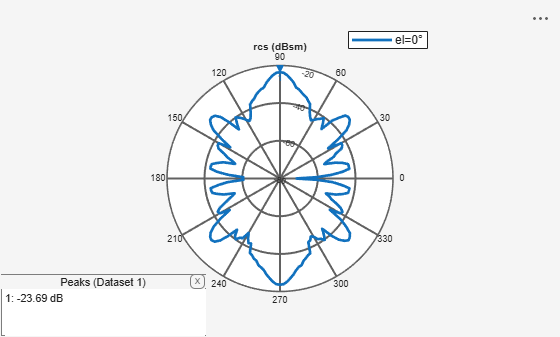Calculate bistatic RCS for a default offset cassegrain antenna at a frequency of 14 GHz.

`S = rcs(cassegrainOffset,14e9,TransmitAngle=[30;60],ReceiveAngle=[30;45])`
```S = -1.9192 ```

## Input Arguments

collapse all

Platform, antenna or array to calculate the RCS, specified as an object.

Example: `platform(FileName="reflector.stl")`

Example: `reflectorParabolic`

Example: `linearArray(Element=dipole)`

Analysis frequency to calculate the RCS, specified as a real-valued scalar in Hz.

Example: `70e6`

Data Types: `double`

Azimuth angles to calculate the RCS, specified as an N-element real vector in degrees.

Example: `90`

Data Types: `double`

Elevation angles to calculate the RCS, specified as an M-element real vector in degrees.

Example: `0:1:360`

Data Types: `double`

### Name-Value Arguments

Specify optional pairs of arguments as `Name1=Value1,...,NameN=ValueN`, where `Name` is the argument name and `Value` is the corresponding value. Name-value arguments must appear after other arguments, but the order of the pairs does not matter.

Example: `CoordinateSystem="polar"`

Before R2021a, use commas to separate each name and value, and enclose `Name` in quotes.

Example: `'CoordinateSystem','polar'`

Coordinate system used to visualize the RCS, specified as a string.

Example: `"rectangular"`

Data Types: `string`

Scale used to visualize or compute the RCS, specified as a string. Use `"log"` scale to calculate and plot the RCS in dBsm unit.

Example: `"linear"`

Data Types: `string`

Transmit and receive wave polarization, specified as a string from one of these transmit-receive combinations:

• `HH` – Horizontal polarized field is transmitted and received.

• `VV` – Vertical polarized field is transmitted and received.

• `VH` – Vertical polarized field is transmitted, and horizontal polarized field is received.

• `HV` – Horizontal polarized field is transmitted, and vertical polarized field is received.

Example: `"VV"`

Data Types: `string`

Flag to enable or disable GPU to perform RCS calculations, specified as either `1` to enable the GPU or `0` to disable.

Example: `1`

Data Types: `logical`

Transmit wave angle, specified as a 2-by-N real matrix representing azimuth and elevation pairs, with each element unit in degrees.

Example: `[30;60]`

Data Types: `double`

Receive wave angle, specified as a 2-by-M real matrix representing azimuth and elevation pairs, with each element unit in degrees.

Example: `[30;60]`

Data Types: `double`

Solver for RCS analysis, specified as a string from these:

• `PO` - Physical Optics

• `MoM` - Method of Moments

• `FMM` - Fast Multipole Method

Example: `"MOM"`

Data Types: `string`

Output type, specified as a string. Specify the type as `"Magnitude"` to calculate and plot the magnitude of RCS values. Specify the type as `"Complex"` to calculate the complex RCS values. Plotting complex RCS values is currently unsupported.

Example: `"Complex"`

Data Types: `string`

## Output Arguments

collapse all

RCS value of the platform, antenna, or array object, returned as an N-by-M real-valued array in dBsm or a complex-valued array depending on the `Type` specified in the input. The size of the array is equal to the number of azimuth values (N) multiplied by the number of elevation values (M).

Data Types: `double`
Complex Number Support: Yes

Azimuth angles of the calculated RCS pattern, returned as an N-element real-valued vector in degrees.

Data Types: `double`

Elevation angles of the calculated RCS pattern, returned as an M-element real-valued vector in degrees.

Data Types: `double`

collapse all

### What Is RCS?

Radar Cross Section (RCS) is the measure of scattering cross section of an object interrogated by a plane wave. The assumption of a plane wave implies that the structure is in the far field of the radiator, which is typically a part of the radar system. RCS is a function of the object's shape, the frequency of the radar, the angle of interrogation of the wave, and the object's material parameters. RCS can also be measured in logarithmic units of dBsm, which is dB relative to a 1 m2 reference area.

RCS is calculated using two typical configurations:

• Monostatic

• Bistatic

By default, the `rcs` function calculates a monostatic RCS. To calculate a bistatic RCS, restrict the `"TransmitAngle"` to 2-by-1.

Monostatic RCS

The monostatic RCS configuration is characterized by a radar system that transmits a signal and receives the backscattered signal from the object being interrogated at the same site. The source of the transmitted electromagnetic waves and the receiving system for the scattered wave are co-located.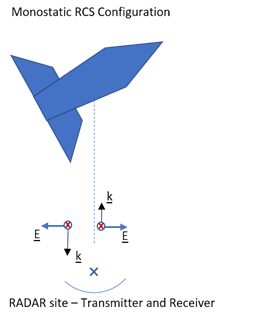Bistatic RCS

In the bistatic RCS configuration, the radar system consists of a fixed radar transmitting site and a fixed or mobile receiving site captures the backscattered waveform from the object.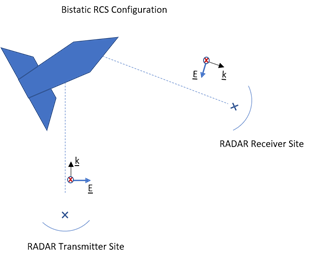### RCS Calculation

RCS is calculated in both a scalar form and a matrix form. Equations for both forms include electric (E) and magnetic (H) field quantities calculated or measured in the far field of the scattering object.

Scalar Form

In the scalar form of RCS, σ is defined as a ratio of the squared backscattered-field to the squared incident field, given by the equation:

`$\sigma =\underset{r\to \infty }{\mathrm{lim}}\text{ }\text{\hspace{0.17em}}4\pi {r}^{2}\frac{{|{E}^{\text{s}}|}^{2}}{{|{E}^{\text{i}}|}^{2}}$`

where Es and Ei represent the scattered and incident electric fields at a specific point in 3-D space.

Matrix Form

The matrix form of the RCS decomposes the incident and the scattered fields into horizontal and vertical polarizations and then computes the ratios of the various combinations between the scattered and incident fields, given by the equation:

`$\left(\begin{array}{cc}{\sigma }_{HH}& {\sigma }_{HV}\\ {\sigma }_{VH}& {\sigma }_{VV}\end{array}\right)=\underset{r\to \infty }{\mathrm{lim}}\text{ }\text{\hspace{0.17em}}4\pi {r}^{2}\left(\begin{array}{cc}\frac{{|{E}_{H}^{\text{s}}|}^{2}}{{|{E}_{H}^{\text{i}}|}^{2}}& \frac{{|{E}_{H}^{\text{s}}|}^{2}}{{|{E}_{V}^{\text{i}}|}^{2}}\\ \frac{{|{E}_{V}^{\text{s}}|}^{2}}{{|{E}_{H}^{\text{i}}|}^{2}}& \frac{{|{E}_{V}^{\text{s}}|}^{2}}{{|{E}_{V}^{\text{i}}|}^{2}}\end{array}\right)$`

where EsH and EiH represent the horizontal polarized components of the scattered and incident electric fields at a given point in 3-D space. EsV and EiV represent the vertical polarized components of the scattered and incident electric fields at a given point in 3-D space.

 Gurel, L., H. Bagrci, J. C. Castelli, A. Cheraly, F. Tardivel. "Validation Through Comparison: Measurement and Calculation of the Bistatic Radar Cross Section of a Stealth Target." Radio Science. Vol. 38, Number 3, 2003, pp.12-1 - 12-8.

 Rao, S.M., D. R. Wilton, A. W. Glisson. "Electromagnetic Scattering by Surfaces of Arbitrary Shape." IEEE Trans. Antennas and Propagation. Vol. AP-30, Number 3, 1982, pp.409-418.

 Jakobus, U., F. M. Landstorfer. "Improved PO-MM Formulation for Scattering from Three-Dimensional Perfectly Conducting Bodies of Arbitrary Shape.." IEEE Trans. Antennas and Propagation. Vol. AP-43, Number 2, 1995, pp.162-169.